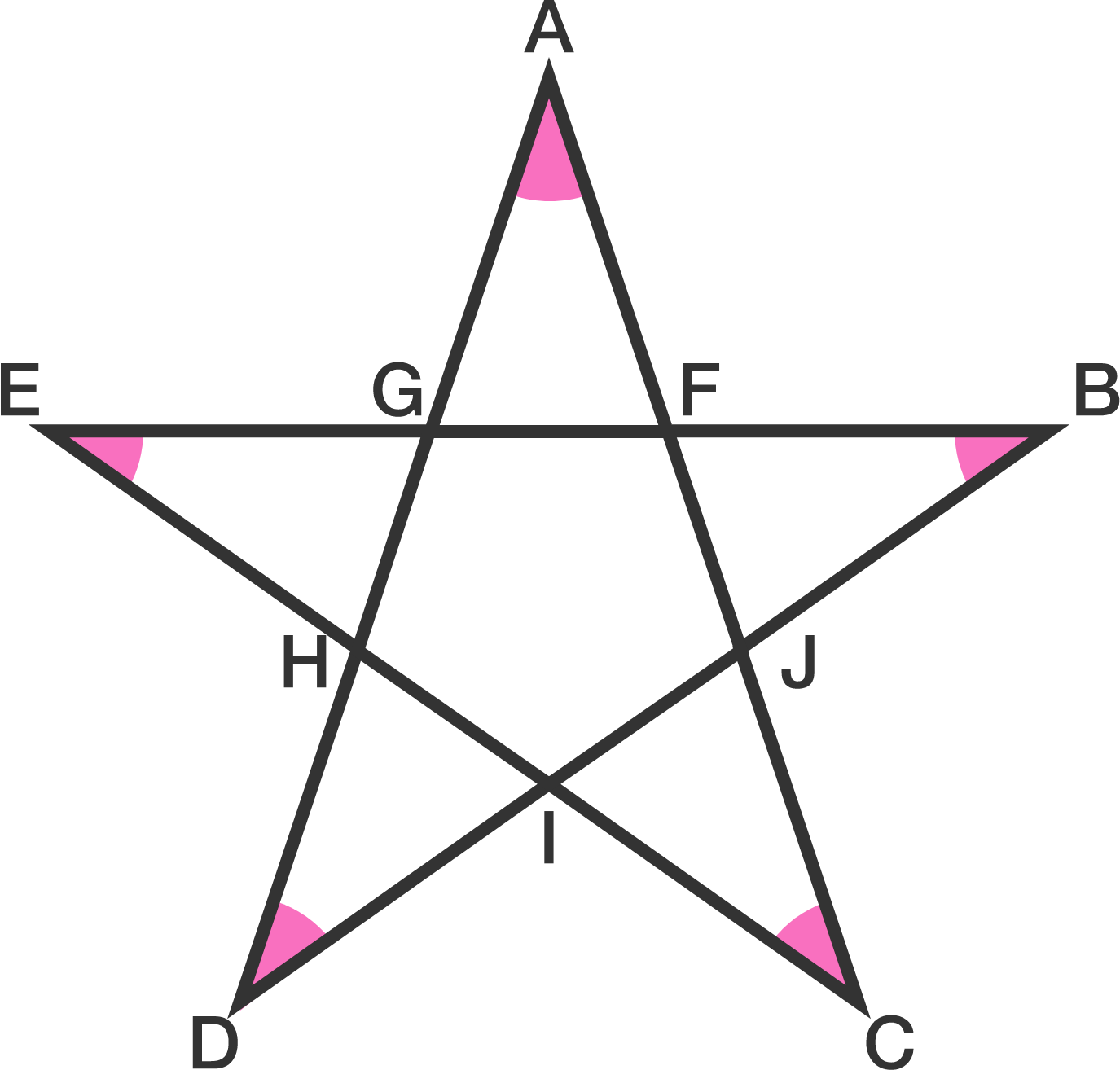# Are You An Angle-Star?

Geometry Level 1If $FGHIJ$ is a regular pentagon, find $\angle A + \angle B + \angle C + \angle D + \angle E \ .$

Details: A regular pentagon is a pentagon with 5 sides of equal length and 5 corner angles of equal measure.

×

Problem Loading...

Note Loading...

Set Loading...